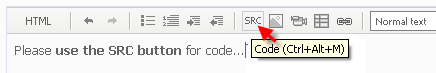16

I am using MQL4's iRSI to code an EA that covers a short when RSI is below 50. The problem is that the cover is triggered before RSI ever reaches 50; the terminal reports that RSI is at 0, while the graph reports RSI at ~60.

Extract of the code:

double currentRSI=iRSI(NULL,0,14,PRICE_CLOSE,0); // the RSI at the current time frame

if (currentRSI<=50)

{

Print("RSI:", currentRSI);

}49

Do not use the value of current bar close, i think that is the problem.

Try this way:

double currentRSI=iRSI(NULL,0,14,PRICE_OPEN,0);

or

double currentRSI=iRSI(NULL,0,14,PRICE_CLOSE,1);170

arobles:

I am using MQL4's iRSI to code an EA that covers a short when RSI is below 50. The problem is that the cover is triggered before RSI ever reaches 50; the terminal reports that RSI is at 0, while the graph reports RSI at ~60.

Extract of the code:

double currentRSI=iRSI(NULL,0,14,PRICE_CLOSE,0); // the RSI at the current time frame

if (currentRSI<=50)

{

Print("RSI:", currentRSI);

}

Indicator value at current open bar always repaints. Use previous bar value for calculation.

```double PreviousRSI=iRSI(NULL,0,14,PRICE_CLOSE,1); // the RSI of previous bar

if (PreviousRSI<=50)

{

Print("Previous bar RSI:", PreviousRSI);

}```3202

LordoftheMoney:

Do not use the value of current bar close, i think that is the problem.

Try this way:

double currentRSI=iRSI(NULL,0,14,PRICE_OPEN,0);

or

double currentRSI=iRSI(NULL,0,14,PRICE_CLOSE,1);

who told u this16

None of the following suggests have worked, but I appreciate the feedback.893

I can see no reason why the posted code would close an order with the RSI value above 50.

I would be looking elsewhere in the code and putting print statements that tell me which line of code closed the order.6884

arobles:
None of the following suggests have worked, but I appreciate the feedback.

Your programming needs to be improved. Looking to your posted EA in CodeBase

And seeing also here your OrderClose function

A few points for you

• Use the SRC button to post your source code• Don't use
`if(OrdersTotal()<1) // check if there are any postions open`

for checking if there are any positions open.....

it will fail if there are manually opened trades on your account or trades from other EA's

Check number of trades with something like

```
if(TotalTrades > 0)    //if(OrdersTotal()<1) no need to check all trades if from EA no opened
{
for(i = OrdersTotal()-1; i >= 0 ; i--)  //count down checking trades
{
if (OrderSelect(i, SELECT_BY_POS, MODE_TRADES)) //Orderselect and check symbol + EAnr
{
if (OrderSymbol()==Symbol() &&  OrderMagicNumber() == MagicNumber)
{
}
}
}
}```

If you set at start of EA TotalTrades > 0 then you will see in the first Orderloop if you have already trades running

• Use extern input for MagicNumber (and Slippage)
• Make your EA checking digitnotation account "the Slippage 3 is for 5 digit notation EURUSD too small"
• `OrderClose(OrderTicket(),OrderLots(),Ask,3,Violet);`
Can be written as
```OrderClose(OrderTicket(),OrderLots(),OrderClosePrice(),Slippage,Violet);
//  For EURUSD  Slippage = 3  when 4 digitnotation
//  For EURUSD  Slippage = 30  when 5 digitnotation
```170

arobles:
None of the following suggests have worked, but I appreciate the feedback.
What error you getting now...??16

Thank you for the coding advice deVries, that will be helpful on this EA too.

Dineshydv, I am not receiving an error. The problem is my trades are being sold too early, because the iRSI function is returning a value of zero instead of the true RSI reading. I changed the way RSI is calculated to PRICE_MEDIAN and it fixed the issue about half the time. Can the inconsistency be due to an erroneous loop that is only triggered on very specific conditions, or could it be a problem with the sample space I am testing?

```/+------------------------------------------------------------------+
//|                                                 Filtered RSI.mq4 |
//|                                                               ar |
//|                                        http://www.metaquotes.net |
//+------------------------------------------------------------------+
extern double TimeFrame=0;
extern double Lots=1;

//+------------------------------------------------------------------+
//| expert initialization function                                   |
//+------------------------------------------------------------------+
int init()
{
//----

//----
return(0);
}
//+------------------------------------------------------------------+
//| expert deinitialization function                                 |
//+------------------------------------------------------------------+
int deinit()
{
//----

//----
return(0);
}
//+------------------------------------------------------------------+
//| expert start function                                            |
//+------------------------------------------------------------------+
int start()
{
//----
// int quick variables
int ticket,total;
double timetocheck=0;

if(OrdersTotal()<1) // check if there are any postions open
{
{

if(AccountFreeMargin()<1000*Lots) // check margin level
{
Print("Free Margin = ",AccountFreeMargin());
return(0);
}
// seting up quick variables for candle stick parts
double currentRSI=iRSI(NULL,0,14,PRICE_MEDIAN,0); // the RSI at the current time frame
double priorRSI=iRSI(NULL,TimeFrame,14,PRICE_MEDIAN,1); // the RSI at two Period prior to current time frame.
double twopriorRSI=iRSI(NULL,TimeFrame,14,PRICE_MEDIAN,2);
double ATR=iATR(NULL,TimeFrame,15,3); // ATR to Filter the ordered trades.

if((priorRSI>=70)&&(currentRSI<70))
{
Print("RSI at short:",currentRSI);
ticket=OrderSend(Symbol(),OP_SELL,Lots,Bid,5,0,0,"BF",16384,0,Green);
if(ticket>0) // if order can be filled
{
{
Print("Time:",OrderOpenTime()); //iy the trader that it was executed.
}
return(0);
}
return(0);
}
//
return(0);
}
return(0);
}

// Setting up fit

timetocheck=TimeCurrent()-OrderOpenTime();

//
if(((3*4*60*60<=timetocheck) && (OrderOpenPrice()-Bid)<3*ATR) ) // sell if the pair has not made progress in the last 12 hours
{
Print("Not enough progress");
}

if (iRSI(NULL,0,14,PRICE_MEDIAN,0)<=50)
{